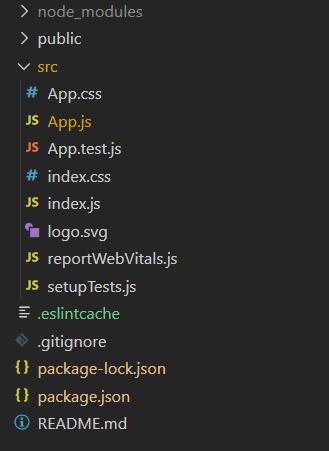# How to set input box to be a floating number in ReactJS ?

• Last Updated : 09 Apr, 2021

If we want to set the input box to be a floating number then we can use the step attribute of the input tag. We have to set the input type as the number and step value by which we want to increase/decrease the floating number. We can also set min and max attributes such that the number will not decrease after the min value and will not increase after the max value.

Creating React Application:

• Step 1: Create a React application using the following command:

`npx create-react-app foldername`
• Step 2: After creating your project folder i.e. foldername, move to it using the following command:

`cd foldername`

Project Structure: It will look like the following.Example:

## App.js

 `import React from ``'react'`` ` `class App extends React.Component {`` ` `  ``state = {``      ``value: 10``  ``}`` ` `  ``onValueChange = (event) => {``      ``this``.setState({value:event.target.value})``  ``}`` ` `  ``render () {``    ``return` `(``      ``
``        ````            `` ``this``.onValueChange(event)}``              ``/>``      ``
``    ``)``  ``}`` ` `}`` ` `export ``default` `App;`

Output:

My Personal Notes arrow_drop_up